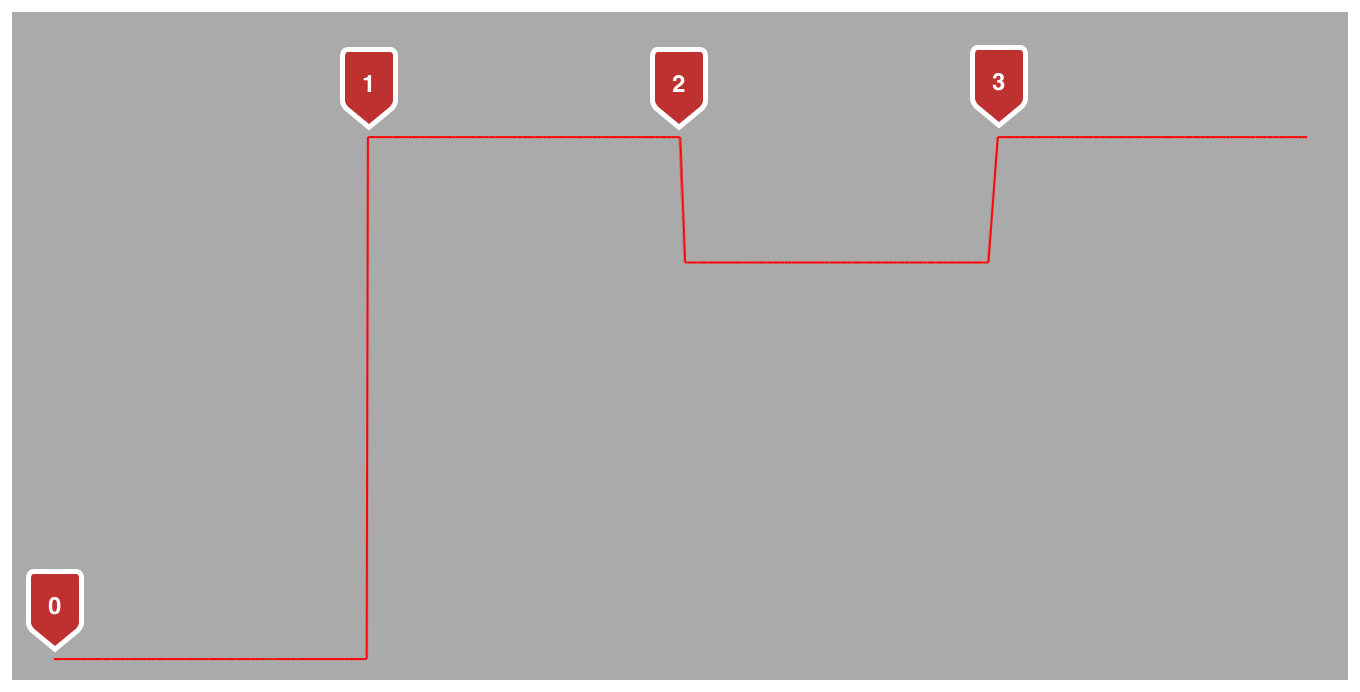Global Variable

# kCAAnimationDiscrete

Each keyframe value is used in turn, no interpolated values are calculated.

## Discussion

Keyframe animations based on discrete calculation interpolation require one less element in the `values` array than the `keyTimes` array. Each `value / keyTime` pair represents the value from the specified time until the next keyframe.

For example, given the `CAKeyframeAnimation` created in Listing 1, the penultimate keyTime, `0.75`, has a related value of `60`. The value of `position.y` will remain at `60` until the animation completes.

A layer animated with the keyframe animation created by the code above and with linearly interpolated horizontal movement would describe a path similar to Figure 1.Figure 1 Tracing the path of an animation using discrete keyframes